• DWA
千次阅读
2022-06-21 00:43:59

来源丨古月居

1.DWA路径规划基本原理

动态窗口法主要是在速度(v,w)空间中采样多组速度，并模拟机器人在这些速度下一定时间(sim_period)内的轨迹。

在得到多组轨迹以后，对这些轨迹进行评价，选取最优轨迹所对应的速度来驱动机器人运动。

该算法突出点在于动态窗口这个名词，它的含义是依据移动机器人的加减速性能限定速度采样空间在一个可行的动态范围内。

2.DWA路径规划流程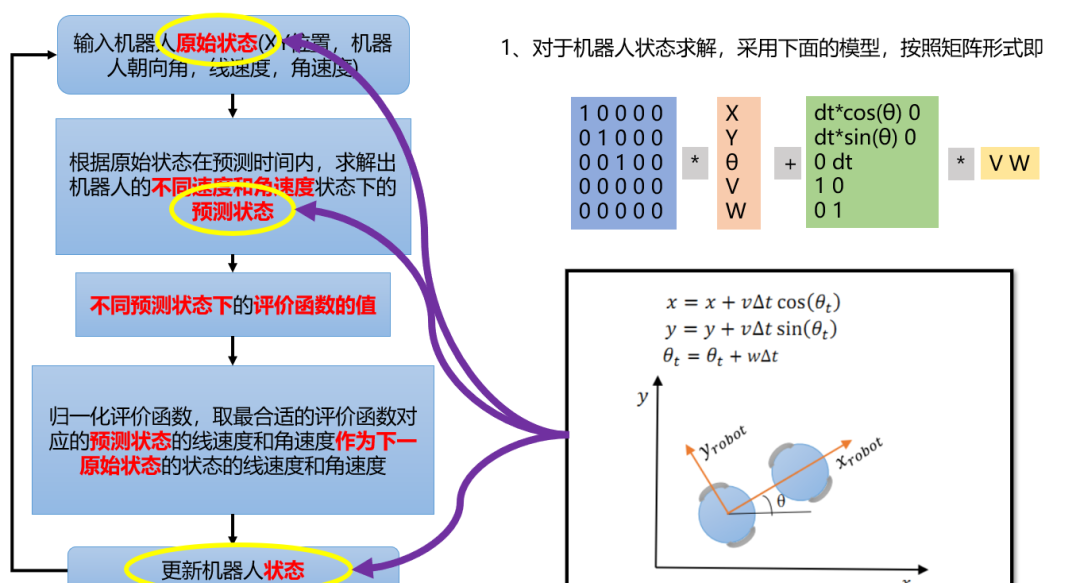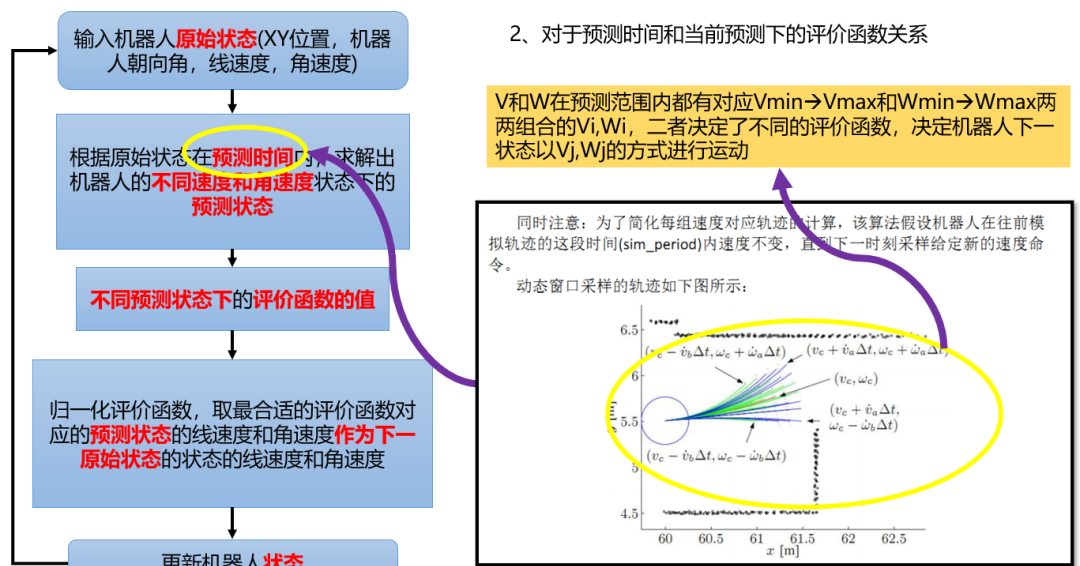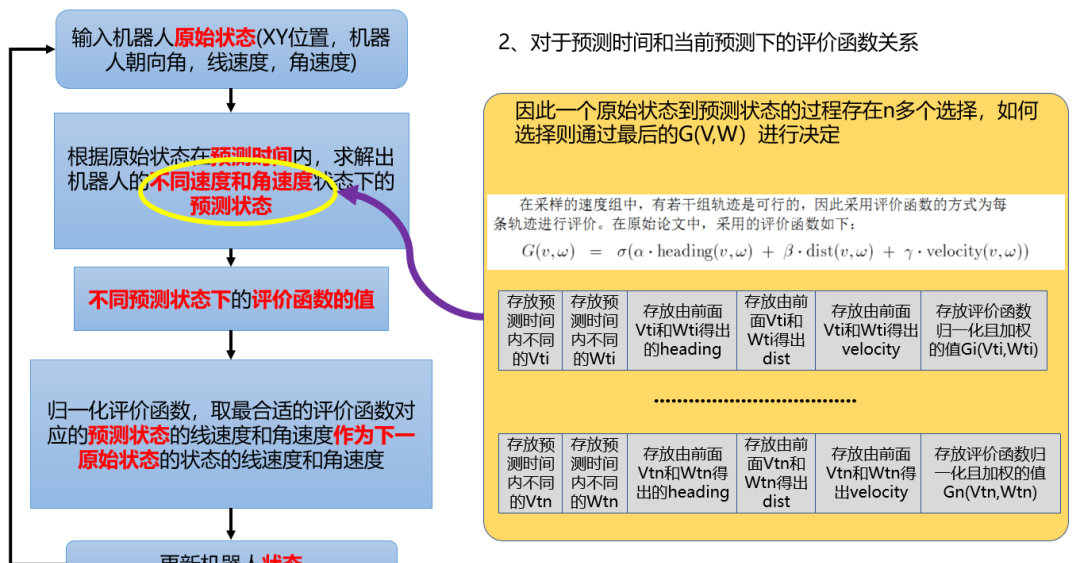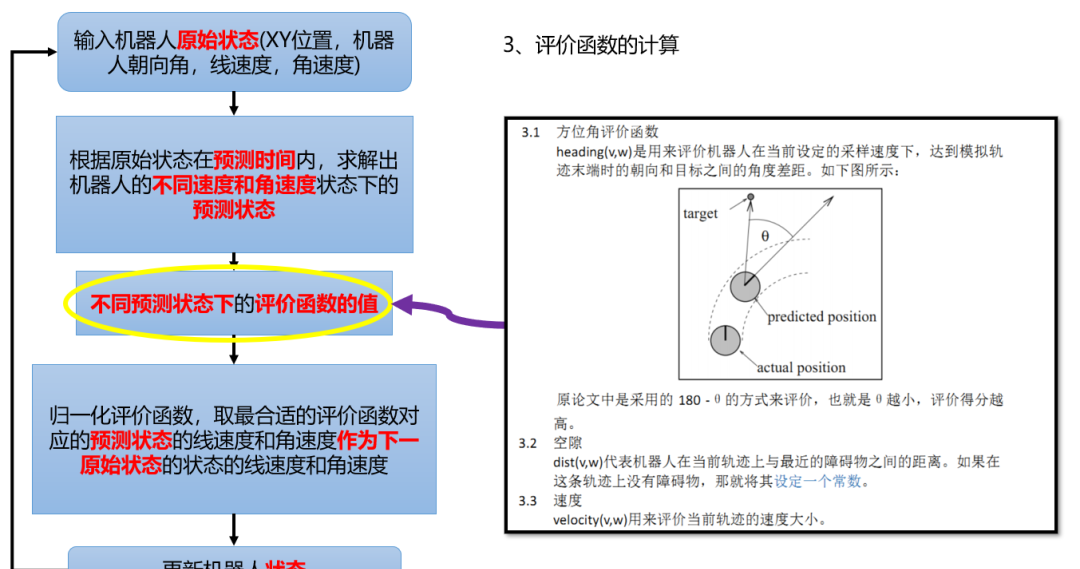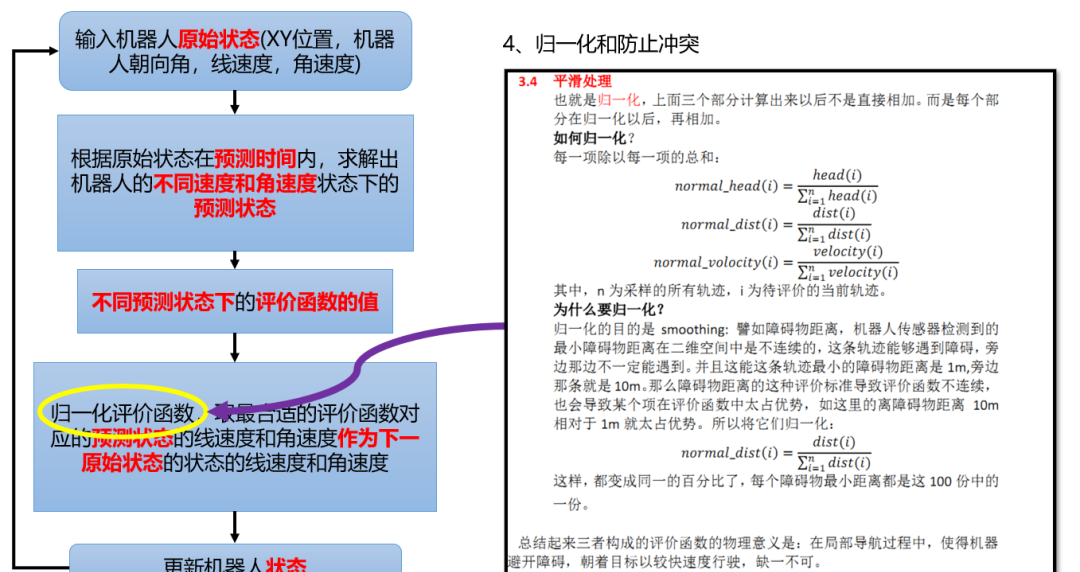3.栅格地图上绘制XY图像

3.1 栅格地图和XY坐标系关系

可以参考：

https://blog.csdn.net/qq_42727752/article/details/117339892

主要区别在于：

• DWA算法是采用XY的坐标值来表示机器人位置和地图特征信息，其实用行列表示是一样的，只是 求解轨迹时，DWA算法路线很细致随机，用行列表示无法表示如[3.21,4.56]这种位置，因此需要进行行列值向坐标值的相互转换

• MATLAB的plot函数和scatter函数等，绘制图像都是针对XY坐标系的

• 在栅格地图上的坐标系X对应于列col，而坐标Y对于与行row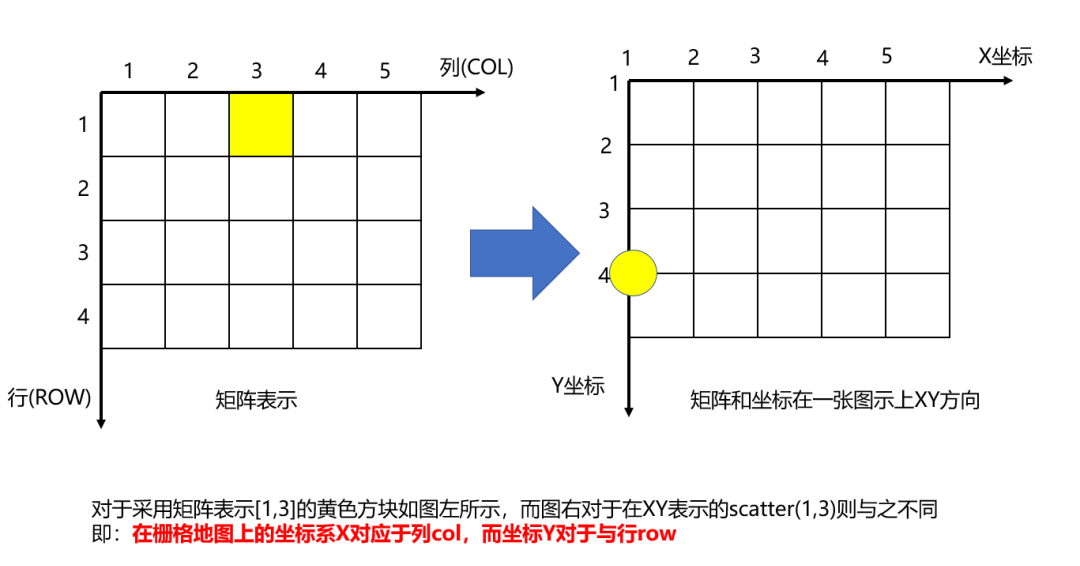3.2 栅格行列位置转坐标系函数sub2coord.m

function coord = sub2coord(sub)
%SUB2COORD 将行列式下标装换为坐标格式，此时的坐标格式和原本认知坐标方向也不一致（如下所示）
%        1 2 3 4 5 6 7 .... X坐标
%      1|——————————>
%      2|
%      3|
%      4|
%      5|
%  Y坐标\/

[l,w] = size(sub);
% 长度l=2表示sub为2*n矩阵
if l == 2
coord(1,:) = sub(2,:);
coord(2,:) = sub(1,:);
end

if w == 2
coord(:,1) = sub(:,2);
coord(:,2) = sub(:,1);
end

end

3.3 栅格坐标系位置转行列位置函数coord2sub.m

function sub = coord2sub(coord)
%COORD2SUB 将坐标转换为矩阵行列格式，坐标格式为下图所示
%        1 2 3 4 5 6 7 .... X坐标
%      1|——————————>
%      2|
%      3|
%      4|
%      5|
%  Y坐标\/

[l,w] = size(coord);
% 长度l=2表示sub为2*n矩阵
if l == 2
sub(1,:) = coord(2,:);
sub(2,:) = coord(1,:);
end

if w == 2
sub(:,1) = coord(:,2);
sub(:,2) = coord(:,1);
end
end

4.DWA路径规划MATLAB代码

tips：本例只有动态障碍物，本例对于sub和coord的转换难理解可以见最后的纯坐标系代码
DWA算法存在陷入局部最优的死循环（可以参考其他文献）

4.1 MATLAB效果示例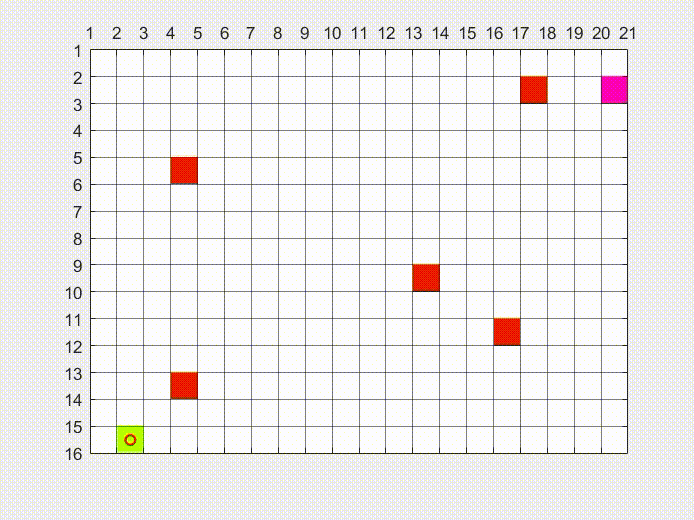4.2 主函数：DWA_sub.m

clear;close all;
rows = 15; cols = 20;                           % 地图行列尺寸
startSub = [15,2];                              % 起点行列位置
goalSub = [2,20];                               % 终点行列位置
dy_obsSub = [4,4; 13,5; 9,14; 2,18; 12,16];     % 动态障碍物行列
step = 0;                                       % 动态障碍物更新运动的频率

% 设置地图属性
field = ones(rows, cols);
field(startSub(1),startSub(2)) = 4;
field(goalSub(1),goalSub(2)) = 5;
dy_obsR = dy_obsSub(:,1);dy_obsC = dy_obsSub(:,2);
dy_obsIndex = sub2ind([rows,cols],dy_obsR,dy_obsC);
field(dy_obsIndex) = 3;
% 颜色表征矩阵
cmap = [1 1 1; ...       % 1-白色-空地
0 0 0; ...           % 2-黑色-静态障碍
1 0 0; ...           % 3-红色-动态障碍
1 1 0;...            % 4-黄色-起始点
1 0 1;...            % 5-品红-目标点
0 1 0; ...           % 6-绿色-到目标点的规划路径
0 1 1];              % 7-青色-动态规划的路径
colormap(cmap);
image(1.5,1.5,field);

% 设置栅格属性
grid on;hold on;
set(gca,'gridline','-','gridcolor','k','linewidth',0.5,'GridAlpha',0.5);
set(gca,'xtick',1:cols+1,'ytick',1:rows+1);
set(gca, 'XAxisLocation','top')
axis image;

% 机器人现在状态
% 对于DWA算法，结算结果建立在XY坐标系上，先将数据转成XY格式
% 坐标系只影响位置，对于机器人其他运动学不影响
robotXY = sub2coord(startSub);
goalCoord = sub2coord(goalSub);
dy_obsCoord = sub2coord(dy_obsSub);

robotT = pi/2;                      % 机器人当前方向角度(0->2pi)
robotV = 0;                         % 机器人当前速度
robotW = 0;                         % 机器人当前角速度
obstacleR=0.6;                      % 冲突判定用的障碍物半径
dt = 0.1;                           % 时间[s]

% 机器人运动学模型
maxVel = 1.0;                       % 机器人最大速度m/s
maxVelAcc = 0.2;                    % 机器人最大加速度m/ss

% 评价系数
alpha = 0.05;                       % 方位角评价系数α
beta = 0.2;                         % 空隙评价系数β
gama = 0.1;                         % 速度评价系数γ
periodTime = 3.0;                   % 预测处理时间,也就是绿色搜寻轨迹的长度

path = [];                          % 记录移动路径

%% 开始DWA算法求解
while true

% 是否到达目的地，到达目的地则跳出循环
if norm(robotXY-goalCoord) < 0.5
break;
end

%% 1、求速度区间==============================================
vel_rangeMin = max(0, robotV-maxVelAcc*dt);
vel_rangeMax = min(maxVel, robotV+maxVelAcc*dt);
rot_rangeMin = max(-maxRot, robotW-maxRotAcc*dt);
rot_rangeMax = min(maxRot, robotW+maxRotAcc*dt);

% 存放当前机器人状态的各个线速度角速度下的评价函数的值
% evalDB格式为n*6，其中6列为下一状态速度、角速度、方向角函数、距离函数、速度函数、评价函数值
evalDB = [];

%% 2、计算不同线速度角速度下的评价函数，目的取出最合适的线速度和角速度******************
for temp_v = vel_rangeMin : 0.01 : vel_rangeMax
for temp_w = rot_rangeMin : pi/180 : rot_rangeMax
%% 2.1 最关键内容，不同线速度角速度都是建立在机器人最初始状态下的
rob_perState = [robotXY(1),robotXY(2),robotT,robotV,robotW]';

%% 2.2 求出在sim_period时间后机器人的状态
for time = dt:dt:periodTime
matE = [1 0 0 0 0;0 1 0 0 0;0 0 1 0 0;0 0 0 0 0;0 0 0 0 0];
matV = [dt*cos(rob_perState(3)) 0;dt*sin(rob_perState(3)) 0;0 dt;1 0;0 1];
% 求解矩阵5*5*5*1+5*2*2*1 = 5*1向量表征机器人假设的状态
% 该模型公式参考模型1，其中dt即△t，利用微分思想即dt是一个很小的数这里均取0.1
rob_perState = matE*rob_perState+matV*[temp_v;temp_w];
end

%% 2.3 计算不同条件下的方位角评价函数,此时机器人状态是在预测时间后的假设状态
% ①方向角评价函数是用来评价机器人在当前设定的采样速度下，
% ②达到模拟轨迹末端时的朝向和目标之间的角度差距
goalTheta=atan2(goalCoord(2)-rob_perState(2),goalCoord(1)-rob_perState(1));% 目标点的方位的角度
evalTheta = abs(rob_perState(3)-goalTheta)/pi*180;

%% 2.4 计算不同条件下的空隙评价函数
% ①空隙评价函数代表机器人在“当前轨迹上”与最近的障碍物之间的距离
% ②如果在这条轨迹上没有障碍物，那就将其设定一个常数
% ③障碍物距离评价限定一个最大值，如果不设定，一旦一条轨迹没有障碍物，将太占比重
dist = inf;
for i=1:length(dy_obsCoord(:,1))
% 利用二范数求距离
disttmp=norm(dy_obsCoord(i,:)-rob_perState(1:2)')-obstacleR;
% 保证dist是最近的障碍物距离
if dist>disttmp
dist=disttmp;
end
end

% 限定距离评价函数不能太大,同时对于没有障碍物的距离函数设置为2倍包容半径
if dist>=2*obstacleR
dist=2*obstacleR;
end

%% 2.5 速度评价函数
% 评价当前轨迹的速度值大小。速度越大，评分越高
velocity = temp_v;

%% 2.6 利用制动距离限定速度是在有效的
% 制动距离的计算，保证所选的速度和加速度不会发生碰撞，参考了博客
stopDist = temp_v*temp_v/(2*maxVelAcc);

% 将有效的速度和角速度存入评价总的评价函数
if dist>stopDist
evalDB=[evalDB;[temp_v temp_w heading dist velocity 0]];
end
end
end

%% 3、对当前状态所有假设的速度加速度组合的评价函数正则化，选取合适的加速度和速度作为下一状态
% 如果评价函数为空则使得机器人停止，即evalDB全0
if isempty(evalDB)
evalDB = [0 0 0 0 0 0];
end

% 将评价函数进行正则化
if sum(evalDB(:,3))~=0
evalDB(:,3)=evalDB(:,3)/sum(evalDB(:,3));
end
if sum(evalDB(:,4))~=0
evalDB(:,4)=evalDB(:,4)/sum(evalDB(:,4));
end
if sum(evalDB(:,5))~=0
evalDB(:,5)=evalDB(:,5)/sum(evalDB(:,5));
end

% 最终评价函数的计算
for i=1:length(evalDB(:,1))
evalDB(i,6)=alpha*evalDB(i,3)+beta*evalDB(i,4)+gama*evalDB(i,5);
end

[~,ind]=max(evalDB(:,6));         % 选取出最优评价函数的索引
nextVR=evalDB(ind,1:2)';          % 机器人下一速度和角速度即为该评价函数对应的速度和角速度

%% 4、选择好角速度线速度更新机器人下一状态
matE = [1 0 0 0 0;0 1 0 0 0;0 0 1 0 0;0 0 0 0 0;0 0 0 0 0];
matV = [dt*cos(rob_perState(3)) 0;dt*sin(rob_perState(3)) 0;0 dt;1 0;0 1];
robot_NextState = matE*[robotXY(1),robotXY(2),robotT,robotV,robotW]'+matV*nextVR;

% 更新状态开启下一轮DWA算法求解
robotXY(1) = robot_NextState(1); robotXY(2) = robot_NextState(2);
robotT = robot_NextState(3); robotV = robot_NextState(4);
robotW = robot_NextState(5);

% 将路径存放到路径矩阵，主要是为了绘图
path = [path;[robotXY(1),robotXY(2)]];

%% 5、绘制图像
field(dy_obsIndex) = 1;
dy_obsSub = coord2sub(dy_obsCoord);
dy_obsR = dy_obsSub(:,1);dy_obsC = dy_obsSub(:,2);
dy_obsIndex = sub2ind([rows,cols],dy_obsR,dy_obsC);
field(dy_obsIndex) = 3;
image(1.5,1.5,field);
scatter(robotXY(1)+0.5,robotXY(2)+0.5,'r','LineWidth',1.5);     % 绘制机器人，红色圆圈表示
plot(path(:,1)+0.5,path(:,2)+0.5,'-b');                                 % 绘制路径
drawnow;

%% 6、将障碍物位置更新实现障碍物也在移动，对于静态障碍物删除从此往下代码
if mod(step,20) == 0
movpos = [0,1; 0,-1; -1,0; 1,0];                 % 对应上下左右四个方向
for i = 1:length(dy_obsCoord(:,1))
temp_obs = dy_obsCoord(i,:);
temp_pos = randi(4);

% 移动范围限制在地图区间里
if dy_obsCoord(i,1) + movpos(temp_pos,1) > 0 && dy_obsCoord(i,1) + movpos(temp_pos,1) < cols
if dy_obsCoord(i,2) + movpos(temp_pos,2) > 0 && dy_obsCoord(i,2) + movpos(temp_pos,2) < rows
dy_obsCoord(i,1) = dy_obsCoord(i,1) + movpos(temp_pos,1);
dy_obsCoord(i,2) = dy_obsCoord(i,2) + movpos(temp_pos,2);
end
end
end
end
step = step + 1;
end

5. DWA算法Python代码

5.1 实现效果

与MATLAB不同，Python代码是绕完所有静态障碍物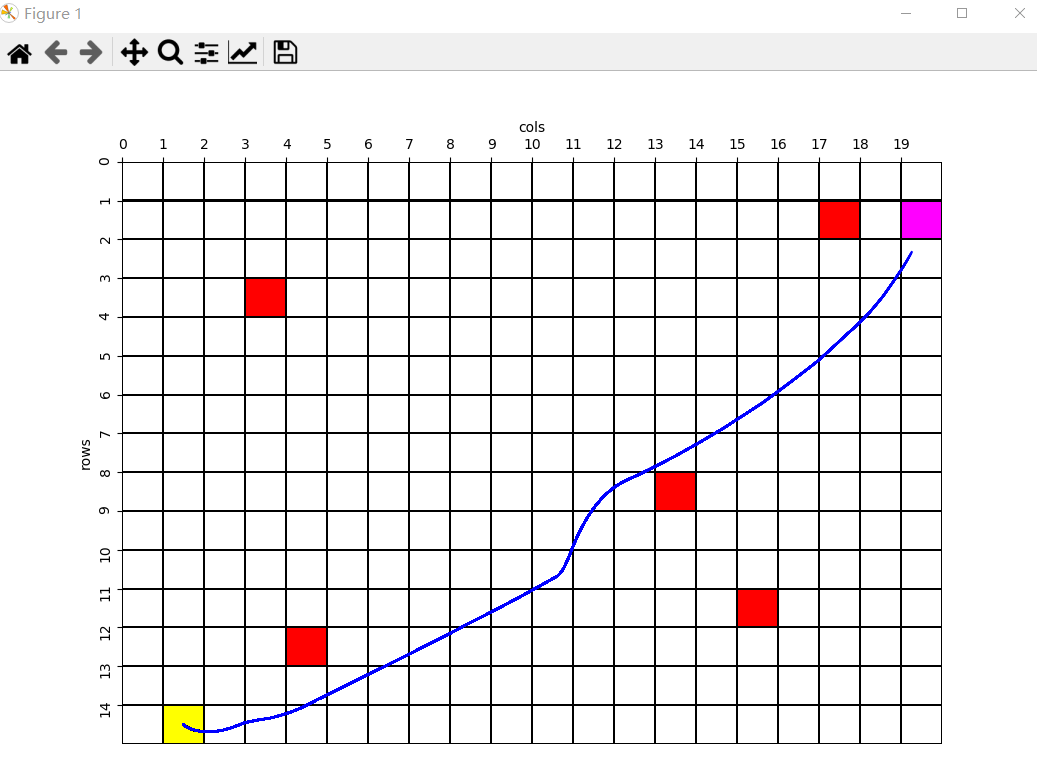5.2 自定义的基本函数PathPlanning.py

参考（1.4.2节）：

https://blog.csdn.net/qq_42727752/article/details/117339892

代码复制重命名即可

5.3 DWA_py.py

import numpy as np
import copy
import matplotlib.pyplot as plt
import PathPlanning

'''
# 设置地图信息，其中rows和cols都是从行列的个数，而非最大行列下标值
# 相比于MATLAB位置从1-->rows，Python的地图信息会相对于数值少1即是0-->rows-1
# 绘制栅格地图的python和MATLAB的坐标系是一样的需要理解转化关系
'''
rows = 15
cols = 20
startSub = [14, 1]
goalSub = [1, 3]
dy_obsSub = [[3, 3], [12, 4], [8, 13], [1, 17], [11, 15]]

field = np.ones([rows, cols])
field[startSub, startSub] = 4
field[goalSub, goalSub] = 5
for i in range(len(dy_obsSub)):
field[dy_obsSub[i], dy_obsSub[i]] = 3

robotXY = PathPlanning.sub2coord(startSub)
goalCoord = PathPlanning.sub2coord(goalSub)

dy_obsCoord = []
for i in range(len(dy_obsSub)):
dy_obsCoord.append(PathPlanning.sub2coord(dy_obsSub[i]))

'''
# 机器人现在状态
# 对于DWA算法，结算结果建立在XY坐标系上，先将数据转成XY格式
# 坐标系只影响位置，对于机器人其他运动学不影响
'''
robotT = np.pi/2
robotV = 0
robotW = 0
obstacleR = 0.6
dt = 0.1

maxVel = 1.0
maxRot = 20.0/180*np.pi
maxVelAcc = 0.2
maxRotAcc = 50.0/180*np.pi

alpha = 0.05
beta = 0.2
gama = 0.1
periodTime = 3.0

path = []
pathx = []
pathy = []
PathPlanning.drawmap(field)

'''
# 代码注释同MATLAB代码是一致的
'''
while True:
# 是否到达目的地，到达目的地则跳出循环
dat = np.sqrt((robotXY-goalCoord)*(robotXY-goalCoord)+(robotXY-goalCoord)*(robotXY-goalCoord))

if dat < 0.5:
break

# 1、求速度区间==============================================
vel_rangeMin = max(0, robotV-maxVelAcc*dt)
vel_rangeMax = min(maxVel, robotV+maxVelAcc*dt)
rot_rangeMin = max(-maxRot, robotW-maxRotAcc*dt)
rot_rangeMax = min(maxRot, robotW+maxRotAcc*dt)

# 存放当前机器人状态的各个线速度角速度下的评价函数的值
# evalDB格式为n*6，其中6列为下一状态速度、角速度、方向角函数、距离函数、速度函数、评价函数值
evalDB = []

## 2、计算不同线速度角速度下的评价函数，目的取出最合适的线速度和角速度******************
for temp_v in np.arange(vel_rangeMin,vel_rangeMax,0.01):
for temp_w in np.arange(rot_rangeMin,rot_rangeMax,np.pi/180):
# 2.1 最关键内容，不同线速度角速度都是建立在机器人最初始状态下的
rob_perState = [robotXY,robotXY,robotT,robotV,robotW]

# 2.2 求出在sim_period时间后机器人的状态
for time in np.arange(dt,periodTime,dt):
matE = np.array([[1,0,0,0,0],[0,1,0,0,0],[0,0,1,0,0],[0,0,0,0,0],[0,0,0,0,0]])
matV = np.array([[dt*np.cos(rob_perState),0],[dt*np.sin(rob_perState),0],[0,dt],[1,0],[0,1]])
# 求解矩阵5*5*5*1+5*2*2*1 = 5*1向量表征机器人假设的状态
# 该模型公式参考模型1，其中dt即△t，利用微分思想即dt是一个很小的数这里均取0.1
rob_perState = np.dot(matE,rob_perState) + np.dot(matV,np.array([temp_v,temp_w]))

#  2.3 计算不同条件下的方位角评价函数,此时机器人状态是在预测时间后的假设状态
#  ①方向角评价函数是用来评价机器人在当前设定的采样速度下，
#  ②达到模拟轨迹末端时的朝向和目标之间的角度差距
goalTheta = np.arctan2(goalCoord-rob_perState,goalCoord-rob_perState)
evalTheta = np.abs(rob_perState-goalTheta)/np.pi*180

# 2.4 计算不同条件下的空隙评价函数
#  ①空隙评价函数代表机器人在“当前轨迹上”与最近的障碍物之间的距离
#  ②如果在这条轨迹上没有障碍物，那就将其设定一个常数
#  ③障碍物距离评价限定一个最大值，如果不设定，一旦一条轨迹没有障碍物，将太占比重
dist = np.inf
for i in range(len(dy_obsCoord)):
disttmp = np.sqrt((dy_obsCoord[i]-rob_perState)*(dy_obsCoord[i]-rob_perState)
+ (dy_obsCoord[i]-rob_perState)*(dy_obsCoord[i]-rob_perState)) - obstacleR

#  保证dist是最近的障碍物距离
if dist > disttmp:
dist = disttmp

# 限定距离评价函数不能太大,同时对于没有障碍物的距离函数设置为2倍包容半径
if dist > 2*obstacleR:
dist = 2*obstacleR

#  2.5 速度评价函数
#  评价当前轨迹的速度值大小。速度越大，评分越高
velocity = temp_v

# 2.6 利用制动距离限定速度是在有效的
#  制动距离的计算，保证所选的速度和加速度不会发生碰撞，参考了博客
stopDist = temp_v*temp_v/(2*maxVelAcc)

# 将有效的速度和角速度存入评价总的评价函数
if dist>stopDist:

# 3、对当前状态所有假设的速度加速度组合的评价函数正则化，选取合适的加速度和速度作为下一状态
#  如果评价函数为空则使得机器人停止，即evalDB全0
if len(evalDB) == 0:
evalDB = [[0,0,0,0,0,0],[0,0,0,0,0,0]]

# 4、将评价函数进行正则化
evalDB = np.array(evalDB)

sum_h = sum(evalDB[:,2])
sum_d = sum(evalDB[:,3])
sum_v = sum(evalDB[:,4])

if sum_h != 0:
for i in range(len(evalDB)):
evalDB[i] = evalDB[i]/sum_h

if sum_d != 0:
for i in range(len(evalDB)):
evalDB[i] = evalDB[i]/sum_d

if sum_v != 0:
for i in range(len(evalDB)):
evalDB[i] = evalDB[i]/sum_v

for i in range(len(evalDB)):
evalDB[i] = alpha*evalDB[i]+beta*evalDB[i]+gama*evalDB[i]

idx = np.argmax(evalDB[:,5])
nextVR = evalDB[idx,0:2]

# 5、选择好角速度线速度更新机器人下一状态
matE = np.array([[1,0,0,0,0],[0,1,0,0,0],[0,0,1,0,0],[0,0,0,0,0],[0,0,0,0,0]])
matV = np.array([[dt*np.cos(rob_perState),0],[dt*np.sin(rob_perState),0],[0,dt],[1,0],[0,1]])
robot_NextState = np.dot(matE,[robotXY,robotXY,robotT,robotV,robotW]) + np.dot(matV,np.array(nextVR))

robotXY = robot_NextState
robotXY = robot_NextState
robotT = robot_NextState
robotV = robot_NextState
robotW = robot_NextState

path.append([robotXY,robotXY])
pathx.append(robotXY+0.5)
pathy.append(robotXY+0.5)

plt.plot(pathx,pathy,'b')
plt.pause(0.01)

5.4 DWA代码纯坐标系版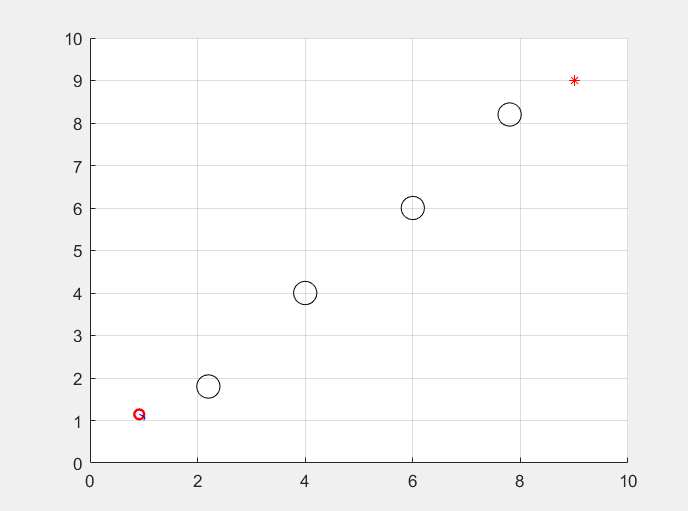clear;
% 机器人现在状态
robotX = 1;                         % 机器人当前X位置
robotY = 1;                         % 机器人当前Y位置
robotT = pi/2;                      % 机器人当前方向角度(0->2pi)
robotV = 0;                         % 机器人当前速度
robotW = 0;                         % 机器人当前角速度

% 地图信息
goal=[9,9];                         % 目标点位置 [x(m),y(m)]
obstacle=[2,2;4,4;6,6;8,8];         % 障碍物位置列表 [x(m) y(m)]
obstacleR=0.6;                      % 冲突判定用的障碍物半径
dt = 0.1;                           % 时间[s]

% 机器人运动学模型
maxVel = 1.0;                       % 机器人最大速度m/s
maxVelAcc = 0.2;                    % 机器人最大加速度m/ss

% 评价系数
alpha = 0.05;                       % 方位角评价系数α
beta = 0.2;                         % 空隙评价系数β
gama = 0.1;                         % 速度评价系数γ
periodTime = 3.0;                   % 预测处理时间,也就是绿色搜寻轨迹的长度

area=[0 10 0 10];                   % 模拟区域范围 [xmin xmax ymin ymax]
path = [];                          % 记录移动路径

%% 开始DWA算法求解
while true

% 是否到达目的地，到达目的地则跳出循环
if norm([robotX,robotY]-goal') < 0.5
break;
end

%% 1、求速度区间==============================================
vel_rangeMin = max(0, robotV-maxVelAcc*dt);
vel_rangeMax = min(maxVel, robotV+maxVelAcc*dt);
rot_rangeMin = max(-maxRot, robotW-maxRotAcc*dt);
rot_rangeMax = min(maxRot, robotW+maxRotAcc*dt);

% 存放当前机器人状态的各个线速度角速度下的评价函数的值
% evalDB格式为n*6，其中6列为下一状态速度、角速度、方向角函数、距离函数、速度函数、评价函数值
evalDB = [];

%% 2、计算不同线速度角速度下的评价函数，目的取出最合适的线速度和角速度******************
for temp_v = vel_rangeMin : 0.01 : vel_rangeMax
for temp_w = rot_rangeMin : pi/180 : rot_rangeMax
%% 2.1 最关键内容，不同线速度角速度都是建立在机器人最初始状态下的
rob_perState = [robotX,robotY,robotT,robotV,robotW]';

%% 2.2 求出在sim_period时间后机器人的状态
for time = dt:dt:periodTime
matE = [1 0 0 0 0;0 1 0 0 0;0 0 1 0 0;0 0 0 0 0;0 0 0 0 0];
matV = [dt*cos(rob_perState(3)) 0;dt*sin(rob_perState(3)) 0;0 dt;1 0;0 1];
% 求解矩阵5*5*5*1+5*2*2*1 = 5*1向量表征机器人假设的状态
% 该模型公式参考模型1，其中dt即△t，利用微分思想即dt是一个很小的数这里均取0.1
rob_perState = matE*rob_perState+matV*[temp_v;temp_w];
end

%% 2.3 计算不同条件下的方位角评价函数,此时机器人状态是在预测时间后的假设状态
% ①方向角评价函数是用来评价机器人在当前设定的采样速度下，
% ②达到模拟轨迹末端时的朝向和目标之间的角度差距
goalTheta=atan2(goal(2)-rob_perState(2),goal(1)-rob_perState(1));% 目标点的方位的角度
evalTheta = abs(rob_perState(3)-goalTheta)/pi*180;

%% 2.4 计算不同条件下的空隙评价函数
% ①空隙评价函数代表机器人在“当前轨迹上”与最近的障碍物之间的距离
% ②如果在这条轨迹上没有障碍物，那就将其设定一个常数
% ③障碍物距离评价限定一个最大值，如果不设定，一旦一条轨迹没有障碍物，将太占比重
dist = inf;
for i=1:length(obstacle(:,1))
% 利用二范数求距离
disttmp=norm(obstacle(i,:)-rob_perState(1:2)')-obstacleR;
% 保证dist是最近的障碍物距离
if dist>disttmp
dist=disttmp;
end
end

% 限定距离评价函数不能太大,同时对于没有障碍物的距离函数设置为2倍包容半径
if dist>=2*obstacleR
dist=2*obstacleR;
end

%% 2.5 速度评价函数
% 评价当前轨迹的速度值大小。速度越大，评分越高
velocity = temp_v;

%% 2.6 利用制动距离限定速度是在有效的
% 制动距离的计算，保证所选的速度和加速度不会发生碰撞，参考了博客
stopDist = temp_v*temp_v/(2*maxVelAcc);

% 将有效的速度和角速度存入评价总的评价函数
if dist>stopDist
evalDB=[evalDB;[temp_v temp_w heading dist velocity 0]];
end
end
end

%% 3、对当前状态所有假设的速度加速度组合的评价函数正则化，选取合适的加速度和速度作为下一状态
% 如果评价函数为空则使得机器人停止，即evalDB全0
if isempty(evalDB)
evalDB = [0 0 0 0 0 0];
end

% 将评价函数进行正则化
if sum(evalDB(:,3))~=0
evalDB(:,3)=evalDB(:,3)/sum(evalDB(:,3));
end
if sum(evalDB(:,4))~=0
evalDB(:,4)=evalDB(:,4)/sum(evalDB(:,4));
end
if sum(evalDB(:,5))~=0
evalDB(:,5)=evalDB(:,5)/sum(evalDB(:,5));
end

% 最终评价函数的计算
for i=1:length(evalDB(:,1))
evalDB(i,6)=alpha*evalDB(i,3)+beta*evalDB(i,4)+gama*evalDB(i,5);
end

[~,ind]=max(evalDB(:,6));         % 选取出最优评价函数的索引
nextVR=evalDB(ind,1:2)';          % 机器人下一速度和角速度即为该评价函数对应的速度和角速度

%% 4、选择好角速度线速度更新机器人下一状态
matE = [1 0 0 0 0;0 1 0 0 0;0 0 1 0 0;0 0 0 0 0;0 0 0 0 0];
matV = [dt*cos(rob_perState(3)) 0;dt*sin(rob_perState(3)) 0;0 dt;1 0;0 1];
robot_NextState = matE*[robotX,robotY,robotT,robotV,robotW]'+matV*nextVR;

% 更新状态开启下一轮DWA算法求解
robotX = robot_NextState(1); robotY = robot_NextState(2);
robotT = robot_NextState(3); robotV = robot_NextState(4);
robotW = robot_NextState(5);

% 将路径存放到路径矩阵，主要是为了绘图
path = [path;[robotX,robotY]];

%% 5、绘制图像
hold off;
scatter(robotX,robotY,'r','LineWidth',1.5);hold on;     % 绘制机器人，红色圆圈表示
plot(goal(1),goal(2),'*r');hold on;                     % 绘制地图终点
scatter(obstacle(:,1),obstacle(:,2),200,'k');hold on;   % 绘制障碍物
plot(path(:,1),path(:,2),'-b');hold on;                 % 绘制路径
axis(area);
grid on;
drawnow;

%% 6、将障碍物位置更新实现障碍物也在移动，对于静态障碍物删除从此往下代码
movpos = [0,0.2; 0,-0.2; -0.2,0; 0.2,0];                 % 对应上下左右四个方向
for i = 1:length(obstacle(:,1))
temp_obs = obstacle(i,:);
temp_pos = randi(4);

% 移动范围限制在地图区间里
if obstacle(i,1) + movpos(temp_pos,1) > 0 && obstacle(i,1) + movpos(temp_pos,1) < 10
if obstacle(i,2) + movpos(temp_pos,2) > 0 && obstacle(i,2) + movpos(temp_pos,2) < 10
obstacle(i,1) = obstacle(i,1) + movpos(temp_pos,1);
obstacle(i,2) = obstacle(i,2) + movpos(temp_pos,2);
end
end
end

end

本文仅做学术分享，如有侵权，请联系删文。

3D视觉工坊精品课程官网：3dcver.com

1.面向自动驾驶领域的多传感器数据融合技术

9.从零搭建一套结构光3D重建系统[理论+源码+实践]

10.单目深度估计方法：算法梳理与代码实现

11.自动驾驶中的深度学习模型部署实战

12.相机模型与标定(单目+双目+鱼眼）

13.重磅！四旋翼飞行器：算法与实战

14.ROS2从入门到精通：理论与实战

15.国内首个3D缺陷检测教程：理论、源码与实战

重磅！3DCVer-学术论文写作投稿 交流群已成立

扫码添加小助手微信，可申请加入3D视觉工坊-学术论文写作与投稿 微信交流群，旨在交流顶会、顶刊、SCI、EI等写作与投稿事宜。

同时也可申请加入我们的细分方向交流群，目前主要有3D视觉CV&深度学习SLAM三维重建点云后处理自动驾驶、多传感器融合、CV入门、三维测量、VR/AR、3D人脸识别、医疗影像、缺陷检测、行人重识别、目标跟踪、视觉产品落地、视觉竞赛、车牌识别、硬件选型、学术交流、求职交流、ORB-SLAM系列源码交流、深度估计等微信群。

一定要备注：研究方向+学校/公司+昵称，例如：”3D视觉 + 上海交大 + 静静“。请按照格式备注，可快速被通过且邀请进群。原创投稿也请联系。▲长按加微信群或投稿▲长按关注公众号

3D视觉从入门到精通知识星球：针对3D视觉领域的视频课程（三维重建系列三维点云系列结构光系列手眼标定相机标定激光/视觉SLAM自动驾驶等）、知识点汇总、入门进阶学习路线、最新paper分享、疑问解答五个方面进行深耕，更有各类大厂的算法工程人员进行技术指导。与此同时，星球将联合知名企业发布3D视觉相关算法开发岗位以及项目对接信息，打造成集技术与就业为一体的铁杆粉丝聚集区，近4000星球成员为创造更好的AI世界共同进步，知识星球入口：

学习3D视觉核心技术，扫描查看介绍，3天内无条件退款圈里有高质量教程资料、答疑解惑、助你高效解决问题

觉得有用，麻烦给个赞和在看~算法 python 机器学习 人工智能 java
更多相关内容
• ## dwa

2021-03-27 05:32:25
• 动态窗口法，可用于局部轨迹规划。手动设置运动学约束和动力学约束信息。
• 路径规划DWA算法精简版，python matplotlib库绘制实时运动信息，注释齐全，简单易懂
• 友讯dwa133无线网卡驱动是一款用来和友讯dwa133无线网卡配合使用的电脑无线网卡驱动软件，给用户带来正常平稳的运行之外，还能够让用户享受极致的上网体验！有兴趣的话欢迎来下载体验！友讯DWA-133无线网卡介绍DWA-...
• ## DWA

2021-02-15 00:17:00
客户项目设置yarn install编译和热重装以进行开发yarn serve编译并最小化生产yarn build整理和修复文件yarn lint自定义配置请参阅。Vue
• DAC 失配噪声将根据通用数据加权平均 (DWA) 传递函数 1±z^(-D) 进行整形，其中 D 是正整数。 DWA 元素选择逻辑计算选择向量。 选择向量根据 DAC 输入选择将使用哪个单元 DAC。 DWA 块主要使用定点块构建。 还...matlab
• 该演示表明，使用动态元件匹配 DAC 编码器 (DWA) 可以将查找表校正系数的数量从 3M 减少到 1，这将使通过数字纠错来缓解 ISI 错误更加可行。 此处演示的校正和校准方法进一步记录在  中。 您需要 Delta-Sigma ...matlab
• 改进的原有代码，修正错误，只需要输入起点和终点即可，并且任意位置都可成功寻路
• D-Link DWA-131 Rev E的官方驱动程序，带有用于使其在较新内核上运行的补丁。 也可以在Rosewill RNX-N180UBE v2 N300无线适配器和TP-Link TL-WN821N V6上使用。 注意：这只是一个“镜像”。 我对此代码或其工作方式...
• dwa动态窗口算法在MATLAB中的仿真代码，有需要的下载。。。。。
• 此文档中是自己整理的关于局部路径规划算法TEB和DWA的相关论文，希望有需求可以读一读，提一句论文是英文的。
• Astar算法和DWA算法的结合实现 main.py：文件可以通过Astar算法实现两点间的路径规划 dwa.py：文件在main.py文件的基础上增加了dwa窗口动态算法，可以实现小车在运行过程中的避障功能 Vplanner.py：dwa算法实现 ...
• dlink dwa192 驱动 2016.2.2 1030.4.1001.2015,dwa192驱动 是针对dwa-192无线网卡而开发的的官方驱动程序，能够适用于Vista/Vista64/WinXP/XP64/Win7/Win7 64/Win8/Win8 64/Win8.1/Win8.1 64/Win10/Win10 64
• DWA171驱动是友讯推出的一款无线网卡的驱动程序，在下载本款驱动可以让你的无线网卡连接更加稳定、更容易被识别，欢迎下载使用！官方简介此软件为DWA-171无线网卡的新版驱动程序，支持Windows8/7、vista及XP系统，仅...
•JavaScript
• 自己写的船舶避碰用的动态避碰仿真，可直接运行，参数可调
• 【路径规划】基于改进动态窗口DWA算法机器人静态避障matlab源码
• 机器人的动态窗口法在matlab上面的认证，还有C++代码和ROS上面的代码。
•算法
• DreamWorks Animation USD插件 该存储库包含用于USD的插件，以及用于支持USD管道的第三方软件的插件。 这些插件由开发和维护。 Hyud与Houdini集成 使用Hydra在查看器中从Pixar的USD导入启用打包的图元，这大大加快了...Python
• 所有模型都在复杂域中使用数据加权平均 (DWA)。 不同的模型有不同的解决方案来实现 DWA。 这些模型用于一项研究，结果论文提交给 IEEE TCAS2： M. Neitola 和 T. Rahkonen，“广义正交数据加权平均”提交给 IEEE ...matlab...